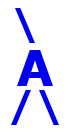## AIRES library reference: ghfpars.

```c
c     Routine directly callable from C/C++ (it could be necessary to
c     append an underscore, "_", to its name depending on the C/C++
c     compiler used).
c
c
subroutine ghfpars(nmax, xmax, x0, lambda, vrb, irc)
c
c     Setting the internal quantities needed to work with the
c     Gaisser-Hillas function related routines.
c     The Gaisser-Hillas function for the profile of charged particles
c     (T. K. Gaisser and A. M. Hillas, in Proc. 15th ICRC (Plovdiv),
c     vol. 8, p. 353 (1977)) is given by:
c
c                      /    X - X0   \ [(Xmax-X0)/lambda]
c        Ngh(X) = Nmax | ----------- |                    *
c                      \  Xmax - X0  /
c
c                          /  Xmax - X  \
c                    * exp | ---------- |
c                          \   lambda   /
c
c     for X > X0 (Ngh(X) = 0 for X < X0).
c
c     Written by: S. J. Sciutto, La Plata 1999, 2000.
c
c
c     Arguments:
c     =========
c
c     nmax, xmax, x0,
c     lambda.......... (input, double precision) The four parameters of
c                      the Gaisser-Hillas function. Xmax, X0, and
c                      lambda are expressed in g/cm2.
c     vrb............. (input, integer) Verbosity control. If vrb is
c                      zero or negative then no error/informative
c                      messages are printed; error conditions are
c                      communicated to the calling program via the
c                      return code. If vrb is positive error messages
c                      will be printed: vrb = 1 means that messages
c                      will be printed even with successful operations.
c                      vrb = 2,3 means that only error messages will
c                      be printed. vrb > 3 is similar to vrb = 3, but
c                      with the additional action of stopping the
c                      program if a fatal error takes place.
c     irc............. (output, integer) Return code. 0 means
c                      successful return.
c
c
c
c<--->
```

 These pages are maintained by Sergio J. Sciutto Back to AIRES library index Back to AIRES home